# Graphing with Functions in Trigonometry: Tutoring Solution Chapter Exam

Exam Instructions:

Choose your answers to the questions and click 'Next' to see the next set of questions. You can skip questions if you would like and come back to them later with the yellow "Go To First Skipped Question" button. When you have completed the practice exam, a green submit button will appear. Click it to see your results. Good luck!

### Page 1

#### Question 4 4. What is the slope of the line tangent to the curve given by the equation y = (x-1)²+2 at the point (1,2)?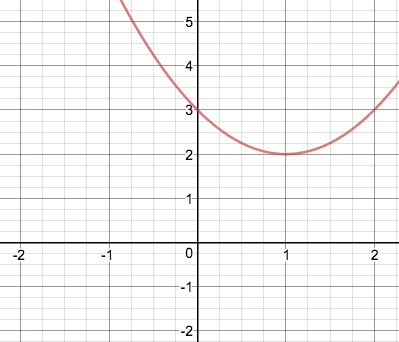### Page 3

#### Question 14 14. The implicit function x^2=y^2 is graphed below. Which functions give the same graph?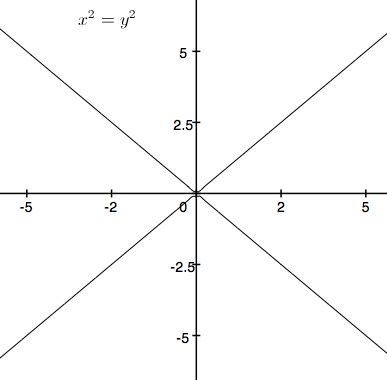### Page 4

#### Question 16 16. If the following graph is an implicit function, then how many real functions would it take to graph it?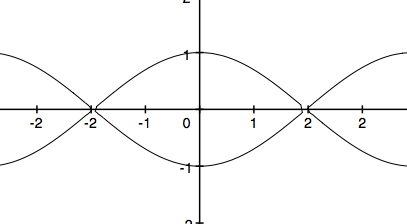#### Question 18 18. How many functions y=f(x) are needed to represent the graph below?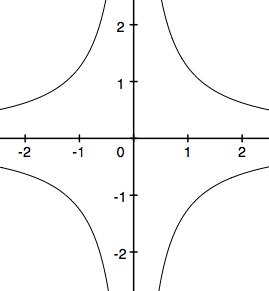### Page 5

#### Question 25 25. What are the asymptotes for this equation?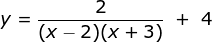### Page 6

#### Graphing with Functions in Trigonometry: Tutoring Solution Chapter Exam Instructions

Choose your answers to the questions and click 'Next' to see the next set of questions. You can skip questions if you would like and come back to them later with the yellow "Go To First Skipped Question" button. When you have completed the practice exam, a green submit button will appear. Click it to see your results. Good luck!

Support